# NCERT solutions for Class 8 Maths chapter 3 - Understanding Quadrilaterals [Latest edition]

#### Chapters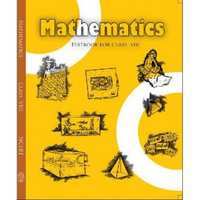Exercise 3.1Exercise 3.2Exercise 3.3Exercise 3.4
Exercise 3.1 [Pages 41 - 42]

### NCERT solutions for Class 8 Maths Chapter 3 Understanding Quadrilaterals Exercise 3.1 [Pages 41 - 42]

Exercise 3.1 | Q 1 | Page 41

Given here are some figures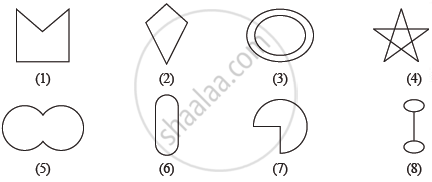Classify each of them on the basis of the following

(a) Simple curve

(b) Simple closed curve

(c) Polygon

(d) Convex polygon

(e) Concave polygon

Exercise 3.1 | Q 2.1 | Page 41

How many diagonals does following have?

Exercise 3.1 | Q 2.2 | Page 41

How many diagonals does following have?

A regular hexagon

Exercise 3.1 | Q 2.3 | Page 41

How many diagonals does following have?

A triangle

Exercise 3.1 | Q 3 | Page 41

What is the sum of the measures of the angels of a convex quadrilateral? Will this property hold if the quadrilateral is not convex? (Make a non-convex quadrilateral and try!)

Exercise 3.1 | Q 4 | Page 41

Examine the table. (Each figure is divided into triangles and the sum of the angles deduced from that.)

 Figure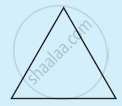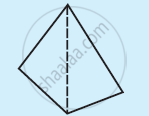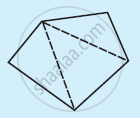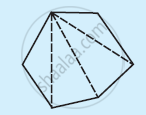Side 3 4 5 6 Angle sum 180° 2 × 180° = (4 − 2) × 180° 3 × 180° = (5 − 2) × 180° 4 × 180° = (6 − 2) × 180°

What can you say about the angle sum of a convex polygon with number of sides?

a) 7

b) 8

c) 10

d) n

Exercise 3.1 | Q 5.1 | Page 42

What is a regular polygon?

Exercise 3.1 | Q 5.2 | Page 42

State the name of a regular polygon of 3 sides

Exercise 3.1 | Q 5.3 | Page 42

State the name of a regular polygon of 4 sides

Exercise 3.1 | Q 5.4 | Page 42

State the name of a regular polygon of 6 sides

Exercise 3.1 | Q 6.1 | Page 42

Find the angle measure x in the given Figure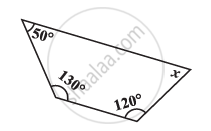Exercise 3.1 | Q 6.2 | Page 42

Find the angle measure x in the given Figure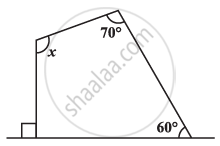Exercise 3.1 | Q 6.3 | Page 42

Find the angle measure x in the given Figure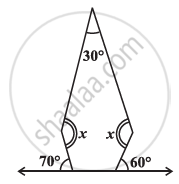Exercise 3.1 | Q 6.4 | Page 42

Find the angle measure x in the given Figure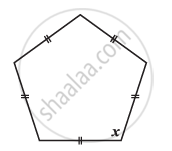Exercise 3.1 | Q 7.1 | Page 42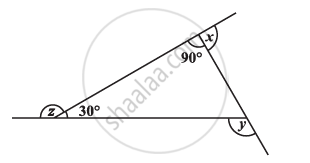Find x + y + z

Exercise 3.1 | Q 7.2 | Page 42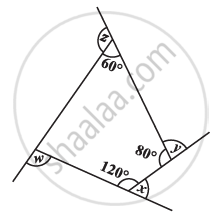Find x + y + z + w

Exercise 3.2 [Page 44]

### NCERT solutions for Class 8 Maths Chapter 3 Understanding Quadrilaterals Exercise 3.2 [Page 44]

Exercise 3.2 | Q 1.1 | Page 44

Find x in the following figures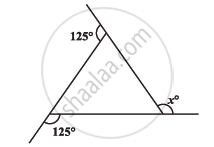Exercise 3.2 | Q 1.2 | Page 44

Find x in the following figures.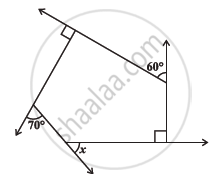Exercise 3.2 | Q 2.1 | Page 44

Find the measure of each exterior angle of a regular polygon of 9 sides

Exercise 3.2 | Q 2.2 | Page 44

Find the measure of each exterior angle of a regular polygon of 15 sides

Exercise 3.2 | Q 3 | Page 44

How many sides does a regular polygon have if the measure of an exterior angle is 24°?

Exercise 3.2 | Q 4 | Page 44

How many sides does a regular polygon have if each of its interior angles is 165°?

Exercise 3.2 | Q 5.1 | Page 44

Is it possible to have a regular polygon with measure of each exterior angle as 22°?

Exercise 3.2 | Q 5.2 | Page 44

Can it be an interior angle of a regular polygon? Why?

Exercise 3.2 | Q 6. (a) | Page 44

What is the minimum interior angle possible for a regular polygon?

Exercise 3.2 | Q 6. (b) | Page 44

What is the maximum exterior angle possible for a regular polygon?

Exercise 3.3 [Pages 50 - 52]

### NCERT solutions for Class 8 Maths Chapter 3 Understanding Quadrilaterals Exercise 3.3 [Pages 50 - 52]

Exercise 3.3 | Q 1 | Page 50

Given a parallelogram ABCD. Complete each statement along with the definition or property used.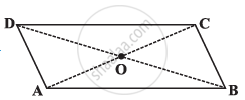(2) ∠DCB = …

(3) OC = …

(4) m∠DAB + m∠CDA = …

Exercise 3.3 | Q 2.1 | Page 51

Consider the given parallelograms. Find the values of the unknowns x, y, z.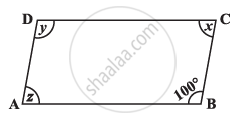Exercise 3.3 | Q 2.2 | Page 51

Consider the given parallelograms. Find the values of the unknowns x, y, z.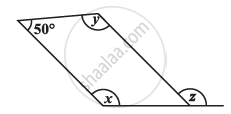Exercise 3.3 | Q 2.3 | Page 51

Consider the given parallelograms. Find the values of the unknowns x, y, z.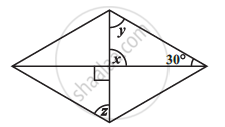Exercise 3.3 | Q 2.4 | Page 51

Consider the given parallelograms. Find the values of the unknowns x, y, z.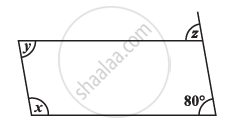Exercise 3.3 | Q 2.5 | Page 51

Consider the given parallelograms. Find the values of the unknowns x, y, z.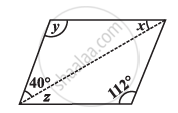Exercise 3.3 | Q 3.1 | Page 51

Can a quadrilateral ABCD be a parallelogram if ∠D + ∠B = 180°?

Exercise 3.3 | Q 3.2 | Page 51

Can a quadrilateral ABCD be a parallelogram if AB = DC = 8 cm, AD = 4 cm and BC = 4.4 cm?

Exercise 3.3 | Q 3.3 | Page 51

Can a quadrilateral ABCD be a parallelogram if ∠A = 70° and ∠C = 65°?

Exercise 3.3 | Q 5 | Page 51

The measures of two adjacent angles of a parallelogram are in the ratio 3:2. Find the measure of each of the angles of the parallelogram.

Exercise 3.3 | Q 6 | Page 51

Two adjacent angles of a parallelogram have equal measure. Find the measure of each of the angles of the parallelogram.

Exercise 3.3 | Q 7 | Page 51

The adjacent figure HOPE is a parallelogram. Find the angle measures xy and z. State the properties you use to find them.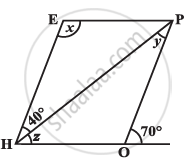Exercise 3.3 | Q 8. (i) | Page 51

The following figures GUNS is parallelograms. Find x and y. (Lengths are in cm)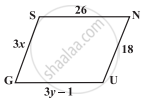Exercise 3.3 | Q 8. (ii) | Page 51

The following figures  RUNS is parallelograms. Find x and y. (Lengths are in cm)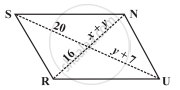Exercise 3.3 | Q 9 | Page 51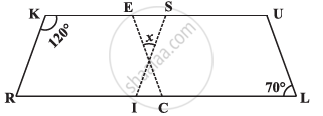In the above figure both RISK and CLUE are parallelograms. Find the value of x.

Exercise 3.3 | Q 10 | Page 52

Explain how this figure is a trapezium. Which of its two sides are parallel?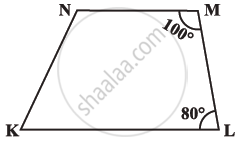Exercise 3.3 | Q 11 | Page 52

Find m∠C in the following figure if bar(AB) || bar(DC)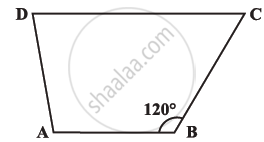Exercise 3.3 | Q 12 | Page 52

Find the measure of ∠P and ∠S, if bar(SP) || bar(RQ) in the following figure. (If you find m∠R, is there more than one method to find m∠P?).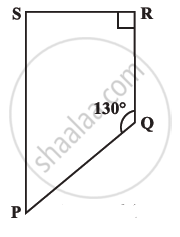Exercise 3.4 [Page 55]

### NCERT solutions for Class 8 Maths Chapter 3 Understanding Quadrilaterals Exercise 3.4 [Page 55]

Exercise 3.4 | Q 1.1 | Page 55

State whether True or False.

All rectangles are squares

• True

• False

Exercise 3.4 | Q 1.2 | Page 55

State whether True or False.

All rhombuses are parallelograms.

• True

• False

Exercise 3.4 | Q 1.3 | Page 55

State whether True or False

All squares are rhombuses and also rectangles.

• True

• False

Exercise 3.4 | Q 1.4 | Page 55

State whether True or False.

All squares are not parallelograms.

• True

• False

Exercise 3.4 | Q 1.5 | Page 55

State whether True or False.

All kites are rhombuses.

• True

• False

Exercise 3.4 | Q 1.6 | Page 55

State whether True or False.

All rhombuses are kites.

• True

• False

Exercise 3.4 | Q 1.7 | Page 55

State whether True or False.

All parallelograms are trapeziums.

• True

• False

Exercise 3.4 | Q 1.8 | Page 55

State whether True or False.

All squares are trapeziums.

• True

• False

Exercise 3.4 | Q 2.1 | Page 55

Identify all the quadrilaterals that have four sides of equal length

Exercise 3.4 | Q 2.2 | Page 55

Identify all the quadrilaterals that have four right angles

Exercise 3.4 | Q 3.1 | Page 55

Explain how a square is a quadrilateral

Exercise 3.4 | Q 3.2 | Page 55

Explain how a square is a parallelogram

Exercise 3.4 | Q 3.3 | Page 55

Explain how a square is a rhombus

Exercise 3.4 | Q 3.4 | Page 55

Explain how a square is a rectangle

Exercise 3.4 | Q 4.1 | Page 55

Name the quadrilaterals whose diagonals  bisect each other

Exercise 3.4 | Q 4.2 | Page 55

Name the quadrilaterals whose diagonals are perpendicular bisectors of each other

Exercise 3.4 | Q 4.3 | Page 55

Name the quadrilaterals whose diagonals are equal

Exercise 3.4 | Q 5 | Page 55

Explain why a rectangle is a convex quadrilateral.

Exercise 3.4 | Q 6 | Page 55

ABC is a right-angled triangle and O is the mid point of the side opposite to the right angle. Explain why O is equidistant from A, B and C. (The dotted lines are drawn additionally to help you)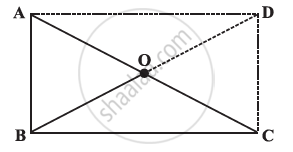Exercise 3.1Exercise 3.2Exercise 3.3Exercise 3.4## NCERT solutions for Class 8 Maths chapter 3 - Understanding Quadrilaterals

NCERT solutions for Class 8 Maths chapter 3 (Understanding Quadrilaterals) include all questions with solution and detail explanation. This will clear students doubts about any question and improve application skills while preparing for board exams. The detailed, step-by-step solutions will help you understand the concepts better and clear your confusions, if any. Shaalaa.com has the CBSE Class 8 Maths solutions in a manner that help students grasp basic concepts better and faster.

Further, we at Shaalaa.com provide such solutions so that students can prepare for written exams. NCERT textbook solutions can be a core help for self-study and acts as a perfect self-help guidance for students.

Concepts covered in Class 8 Maths chapter 3 Understanding Quadrilaterals are Properties of Trapezium, Angle Sum Property of a Quadrilateral, Properties of Kite, Classification of Polygons, Properties of a Parallelogram, Concept of Curves, Different Types of Curves - Closed Curve, Open Curve, Simple Curve., Concept of Polygons - Side, Vertex, Adjacent Sides, Adjacent Vertices and Diagonal, Interior Angles of a Polygon, Exterior Angles of a Polygon and Its Property, Concept of Quadrilaterals - Sides, Adjacent Sides, Opposite Sides, Angle, Adjacent Angles and Opposite Angles, Property: The diagonals of a rhombus are perpendicular bisectors of one another., Properties of Rhombus, Property: The Opposite Sides of a Parallelogram Are of Equal Length., Property: The Opposite Angles of a Parallelogram Are of Equal Measure., Property: The adjacent angles in a parallelogram are supplementary., Property: The diagonals of a parallelogram bisect each other. (at the point of their intersection), Property: The Diagonals of a Rectangle Are of Equal Length., Properties of Rectangle, Properties of a Square, Property: The diagonals of a square are perpendicular bisectors of each other..

Using NCERT Class 8 solutions Understanding Quadrilaterals exercise by students are an easy way to prepare for the exams, as they involve solutions arranged chapter-wise also page wise. The questions involved in NCERT Solutions are important questions that can be asked in the final exam. Maximum students of CBSE Class 8 prefer NCERT Textbook Solutions to score more in exam.

Get the free view of chapter 3 Understanding Quadrilaterals Class 8 extra questions for Class 8 Maths and can use Shaalaa.com to keep it handy for your exam preparation